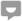# Degrees of Price Elasticity of Demand

Knoji reviews products and up-and-coming brands we think you'll love. In certain cases, we may receive a commission from brands mentioned in our guides. Learn more.
Degrees of Price Elasticity of Demand A change in price leads to a change in demand. If price increases, demand contracts, and vice versa. But variation in demand is not always uniform when there is a change in price. In some cases, with a given change i

Degrees of Price Elasticity of Demand

A change in price leads to a change in demand. If price increases, demand contracts, and vice versa. But variation in demand is not always uniform when there is a change in price. In some cases, with a given change in price, demand shows a marked change, whereas in other cases the demand is not so responsive to changes in price.

For example, even if the price of salt varies widely, we continue to buy almost the same quantity the demand is inelastic. But if the price of wireless sets falls, many people, who could not afford before, may now be induced to buy; the demand will stretch or expand; it is elastic.

Depending upon the degree of responsiveness of the amount demanded to the price changes, the economists distinguish five kinds of price elasticity of demand.

1. Perfectly Elastic Demand- When even an infinitesimally small reduction in price leads to an unlimited extension of demand and an infinitesimally small rise in the price cause the demand to fall to zero, we say that the demand is perfectly elastic or the elasticity of demand is infinite.

2. Perfectly Inelastic Demand-When, however - much the price may fall or rise, the amount demanded remains the same, i.e., the demand is non-responsive to price changes, we say that the demand is perfectly inelastic or the elasticity of demand is zero.

It may, however, be pointed out that both absolutely inelastic demand and infinitely elastic demand are the two limits which are seldom met with in real life and can only be conceived of theoretically. On the other hand, in actual life we come across elasticity of demand which is somewhere between these two limits, i.e., it is more than zero but less than infinity.

3. Relatively Inelastic Demand- When a large change in the price of a commodity produces a less than proportionate change in the quantity demanded, we say that the demand is relatively inelastic. Alternatively, it is also referred to as elasticity of demand less than unity.

4. Unitary Elasticity- When a proportionate change in price brings about an equal and proportionate change in the demand, we say that the demand has Unitary Elasticity. A demand, Curve having unitary elasticity is called an equilateral or rectangular hyperbola.

5. Relatively Elastic Demand – When a small change in the price is accompanied by a more proportionate change in the quantity demanded, we say that the demand is relatively elastic. Alternatively, it is said that the elasticity of demand is greater than unity.

## 1 commentGuest
Posted on Jun 18, 2011